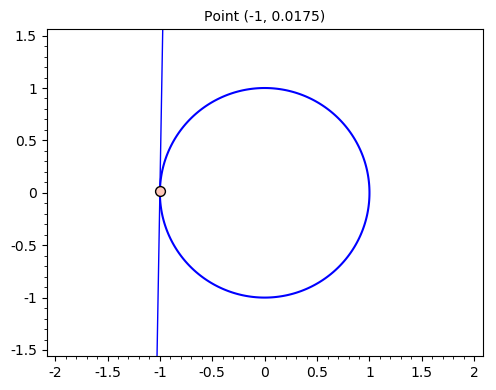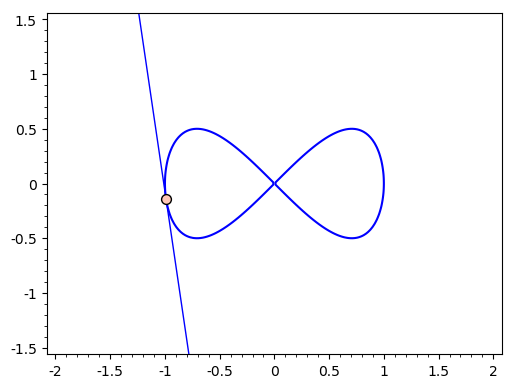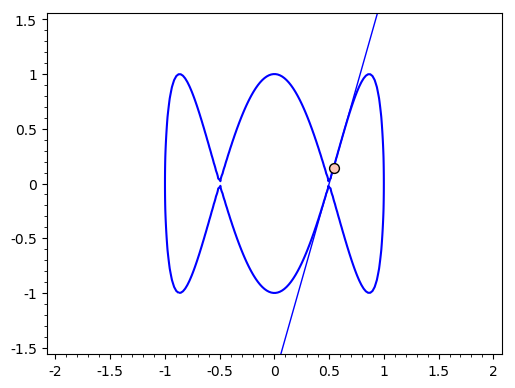# Example 1: Circle¶

$\Large x^2 + y^2 = 1$

In :
# Now pick a point a and b on the curve, using the fact that we know this is the unit circle
t = 179*pi/180
a = cos(t)
b = sin(t)

# First plot the relation
var("x y")
p1 = implicit_plot(x^2 + y^2 - 1, (x,-1.5,1.5), (y,-1.5,1.5), title="Point (%.3g, %.3g)"%(N(a), N(b)))

# Apply the fact that the tangent line is l(x) = b + m*(x-a), where m is -a/b
l = b + (-a/b)*(x-a)
p2 = plot(l, (x, -2, 2), ymin=-1.5, ymax=1.5)
p3 = scatter_plot([[a, b]])

show(p1+p2+p3)# Example 2: Figure 8¶

$\Large x^2 = x^4 + y^2$

In :
# First plot the relation
var("x y")
p1 = implicit_plot(x^4 - x^2 + y^2, (x,-1.5,1.5), (y,-1.5,1.5))

# Now pick a point a and b on the curve,
# using the fact that this can be parameterized by (cos(t), sin(2t)/2)
t = 3
a = cos(t)
b = sin(2*t)/2

# Apply the fact that the tangent line is l(x) = b + m*(x-a), where m is (a-2a^3)/b
mtan = (a-2*a^3)/b
l = b + mtan*(x-a)
p2 = plot(l, (x, -2, 2), ymin=-1.5, ymax=1.5)
p3 = scatter_plot([[a, b]])

show(p1+p2+p3)# Example 3: ABC Australia Logo¶

$\Large (4x^3 - 3x)^2 + y^2 = 1$

In :
p1 = implicit_plot((4*x^3-3*x)^2+y^2-1, (x,-1.5, 1.5), (y,-1.5,1.5))

# Now pick a point a and b on the curve,
# using the fact that this can be parameterized by (cos(t), sin(3t))
t = 1
a = cos(t)
b = sin(3*t)

# Find the tangent line
mtan = -((4*a^3-3*a)*(12*a^2-3)/b)
l = b + mtan*(x-a)
p2 = plot(l, (x, -2, 2), ymin=-1.5, ymax=1.5)
p3 = scatter_plot([[a, b]])

show(p1+p2+p3)In [ ]: